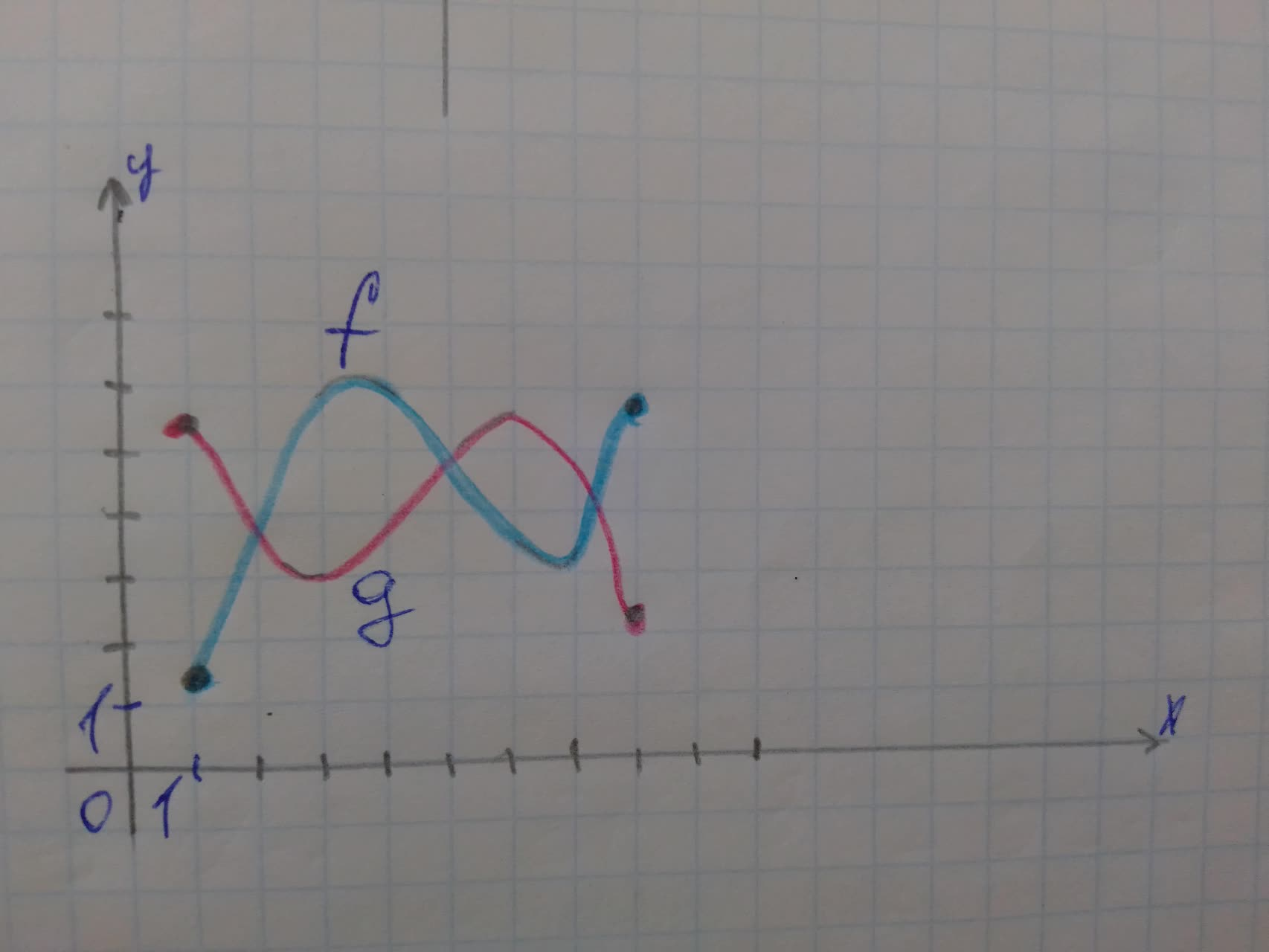# Graphs of the functions f and g are given. a) Which is larger, f(6) or g(6)?Maaghu 2021-11-21 Answered
Graphs of the functions f and g are given.
a) Which is larger, f(6) or g(6)?
b) Which is larger, f(3) or g(3)?
c) Find the values of x for which $$\displaystyle{f{{\left({x}\right)}}}={g{{\left({x}\right)}}}$$.• Questions are typically answered in as fast as 30 minutes

### Plainmath recommends

• Get a detailed answer even on the hardest topics.
• Ask an expert for a step-by-step guidance to learn to do it yourself.Fearen
a) $$\displaystyle{f{{\left({6}\right)}}}={3.5}$$ and $$\displaystyle{g{{\left({6}\right)}}}={5.5}$$
Clearly g(6) is larger.
b) $$\displaystyle{f{{\left({3}\right)}}}={6}$$ and $$\displaystyle{g{{\left({3}\right)}}}={3}$$
Clearly f(3) is larger.
c) From the graph we can see that the two curves meets at $$\displaystyle{x}={2}$$ and $$\displaystyle{x}={7}$$
Hence, $$\displaystyle{f{{\left({x}\right)}}}={g{{\left({x}\right)}}}$$ when $$\displaystyle{x}={2},{7}.$$